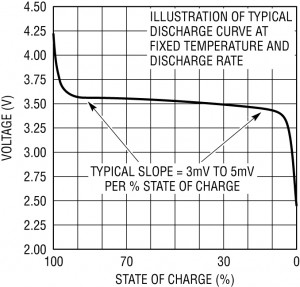# LoPy with Expansion board, How do you measure LiPo Battery up to 4.2V?

• I am wrapping my head around measuring the battery voltage on pin 16 with the Attn set to '3'. My understanding is that pin 16 with the resistors will output a voltage of 0 to 1.4 that can be interpreted as a voltage from 0 to 3.9V in 4096 steps (attn=3). The BQ24040 on the expansion board (from TI) charges Li-ion batteries with a min and max charge voltage of 4.2V.

Maybe this is more of a math problem with a simple solution.

I am using protected 18650 batteries so they are not left to undercharge by accident, low end Voltage cut off is around 3V I think(haven't tested) and the high end at 4.2V.

If the range is 3V (0%) to 3.9V(100%), there is a 0.9V range of juice. The battery percentage would look something like:

``````percent_battery = ((meanADC/4096*3.548/0.3275)-3)/0.9
``````

If I want to show a battery charged at 100% (at 4.2 volts) is that possible with the LoPy and expansion board?

Any feedback is appreciated.

• @jmarcelino
Once again, thanks for the great feedback. I was looking for a simple way to alert when the battery needs to be recharged. Looks like some simple logic could be: if voltages gets to 3.3, alert for recharge...

So many LiPo batteries have differing characteristics, even among the same model/make/capacity/manufacturer. I don't think there is real value is tracking a percentage... without extensive battery testing. Simplest thing to do is just report voltage.

From the example from HERE do you recommend using the following:

``````numADCreadings = const(100)
i = 0
i += 1
``````

From the ACD pin 16 voltage, the voltage is the meanACD*1400/1024
Does not seem right, the output is 4966.3634.

• The other thing is lithium batteries are notoriously difficult to estimate in percentage. You wouldn't believe some of complexity of the algorithms the industry uses to make this appear as a nice and easy value for the user.

Take this typical LiPo discharge curve for exampleYou'll see that it's not linear, from about 90% to 5% the voltage remains almost const and only changes by about 3-5mV per %.

A simple formula like the one you posted would show the battery quickly falling to 50%, remaining around there for a very long time and then when finally dropping to around 40% would mean in reality the device was almost about to die.

• @ledbelly2142
First I think it helps if you use the attenuation constants, e.g. ADC.ATTN_11DB instead of arbitrary meaningless numbers like 3.

With ADC.ATTN_11DB the full range of the ADC is 3.9v so ADC reading 0 mean 0 volts and 4095 means 3.9v (but this is just a numeric range, don't feed it over 3.3V!)

The voltage divider on the expansion board "divides" the input voltage so that if the battery is at 4.2v you see ~ 1.4v into the ADC. This means the maximum ADC value - at ATTN_11DB - you would ever read for the battery (when at 4.2v) is 1.4 * 4095 / 3.9 = 1470.

This isn't very good because you're only using 1/3 of the range of the of the ADC , so I'd suggest using a lower attenuation.